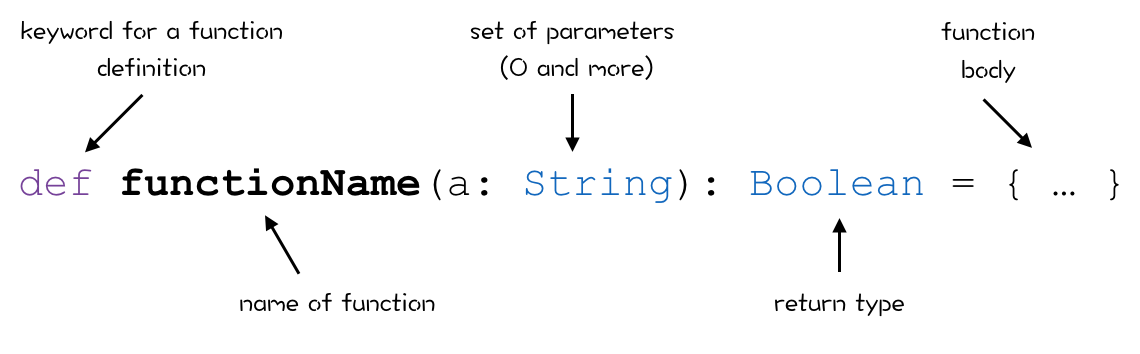# Scala: Introduction to Functions

### Here's an intro to Scala functions, with a look at function syntax, and in-depth examples.

· Java Zone · Analysis
Save
9.89K Views

alex_zvolinskiy scala combines two programming paradigms: object oriented and functional. as a result you get the best from the two worlds. from the one side you can describe models as objects, and from the other side you can apply functions to them. and as you may guess, it’s really awesome! let’s discover the basics of scala functions.

every time you need to perform some business logic or a simple action, start writing a function. with the help of functions you can do almost everything. why only “almost”? because to describe a model you still need use classes and objects.

## function syntax

functions can work with arguments and perform actions with them. functions can return values. you can make a composition from multiple functions in order to complete one complex task, and all this is wrapped in a flexible syntax. so how do we create a function in scala? here is what a simple function declaration looks like:

``````
def welcomeuser(name: string): unit = {
println("hello, "+name)
}
``````

this function prints a greeting. the greeting contains a name which is passed to the ``` welcomeuser ``` function as a parameter. and what about that weird word ``` unit ``` in the first line? it indicates that the function doesn’t return anything.

after a function is declared we can use it. in order to call a function we need to write its name and pass parameters into it.

``````
welcomeuser("alex")
//the output is "hello, alex"
``````

here is another example of a scala function :

``````def iseven(number: int): boolean = {
if (number % 2 == 0)
true
else
false
}``````

the function above accepts an integer as a parameter and returns a boolean value. it’s logic is pretty trivial - it checks if the number argument is even or odd.

here is a general pattern for a function declaration in scala:at first you need to write a ``` def ``` keyword. the next step is to write a function name. if the function needs some arguments you write them in the brackets in a format ``` name: type ``` , otherwise brackets remain empty. then you specify a return type of the function. and finally you write a function body.

## more function examples

now let’s consider several more examples of functions. we can start from the pretty useless function, which doesn’t has any arguments and prints some string:

``def uselessprint(): unit = { println("ho-ho-ho") }``

what about a function which takes more than one parameter?

``````def taxcalculation(amount: double, tax: int): double = {
(amount / 100) * tax
}``````

in the example above we create the ``` taxcalculation(amount: double, tax: int) ``` function, and it accepts two parameters and calculates taxes based on them. pay attention to the function body. its last line has a ``` double ``` type (after calculation). a type of the last line (last expression) in the method is a type which a function returns.
here is what this function looks like in the repl:scala allows us to omit a return type in a function declaration. but scala knows what function returns due to type inference . we have discussed the type inference in the previous post about variables.

``````def stringcutter(str: string, length: int) = {
val result = str.substring(0, length)
result
}``````

here is what happens in the repl when we declare this function and use it:there are a lot of syntax elements which can be omitted when you are working with functions in scala, e.g. curly brackets if a function body suits well for a single line. let’s rewrite the ``` taxcalculation(amount: double, tax: int) ``` :

``def taxcalculation(amount: double, tax: int) = (amount / 100) * tax``

## functions under a microscope

as was said in the previous post, everything in scala is an object. functions are not exception. so actually, all functions which we have demonstrated in the current article are objects. scala has some sort of abstractions for functions depends on number of arguments, starting from 0 (function without arguments) to 22 (yeah, imagine an ugly function with 22 parameters!).

using these abstractions you can also define functions, but it’s not an elegant way - just look at this:

``````val taxcalculation = new function2[double, int, double] {
def apply(amount: double, tax: int): double = (amount/100)*tax
}``````

this is the absolute equivalent of the function which calculates taxes in the previous paragraph. this code sample is provided just for demonstration of the functions nature - they are objects. here is how it looks like in the repl:## summary

functions in scala are the smallest building blocks for business logic. they are really powerful and can be used in many situations for different purposes. in this post we overviewed the basics of functions and their syntax. but there are a lot of things which you need to know about them, e.g. what is function composition or how to pass functions as an argument to another function.

Topics:
scala, functional programming

Published at DZone with permission of Alexey Zvolinskiy, DZone MVB.

Opinions expressed by DZone contributors are their own.